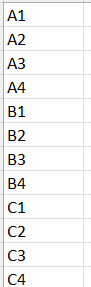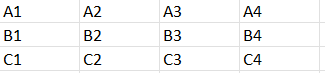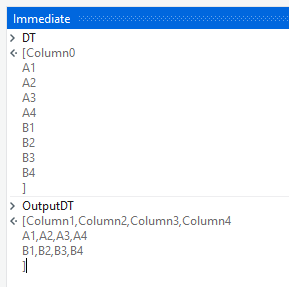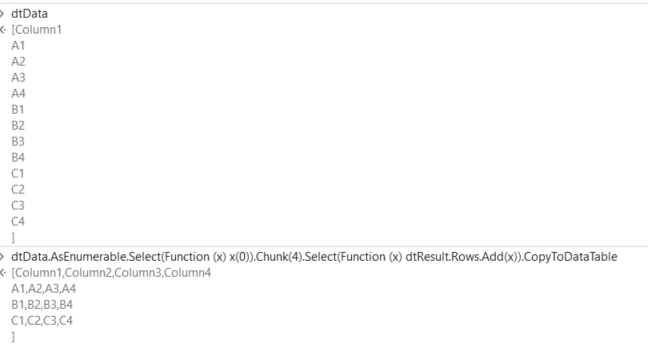# Uipath Transpose excel after every 4 row

Hi All,

I need to transpose after every 4 row.

From:To:Thank you!

Hi @Shawn11 ,

Could you try with the below Steps :

1. Prepare a Datatable using `Build Datatable` activity with 4 Columns as we need number of columns as 4, let is be named as `OutputDT`.

2. Next, Read the Excel sheet as a Datatable without `Add Headers` enabled, let the output datatable be named as `DT`.

3. We could then use the below Expression in an `Assign` activity.

``````OutputDT = Enumerable.Range(0,CInt(Math.Ceiling(DT.Rows.Count/4))).Select(Function(x)OutputDT.Rows.Add(DT.AsEnumerable.Skip(x*4).Take(4).Select(Function(y)y(0).ToString).ToArray)).CopyToDatatable
``````
1. Next, we can write the `OutputDT` to an Excel sheet without `Add Headers` being enabled.

From Debug Panel :1 Like

Lets assume

• 4 is fixed
• Project is set to Windows Compatibility
• Excel is read in into a datatable - dtData

Prepare an empty DataTable with Build DataTable Activity, Configure the 4 cols - dtResult

Assign Activity
dtResult =

``````dtData.AsEnumerable.Select(Function (x) x(0)).Chunk(4).Select(Function (x) dtResult.Rows.Add(x)).CopyToDataTable
``````3 Likes

Hi Sir,

I got this error,